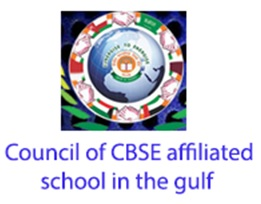Register for Maths, Science, English, Reasoning Olympiad Exams Register here | Book Free Demo for Live Olympiad Classes here | Check Olympiad Exam Dates here | Buy Practice Papers for IMO, IOM, HEO, IOEL etc here | Login here to participate in all India free mock test on every Saturday## Parent Organisation:

Council of CBSE Schools in Gulf

Not Available.

## Syllabus:

For Class X-

UNIT-1 Number System

1. Real Numbers.

UNIT-2 Algebra

1. Polynomials
2. Pair of Linear Equations in Two Variables
1. Arithmetic Progressions

UNIT-3 Geometry

1. Triangles
2. Circles
• Constructions

UNIT-4 Statistics and Probability

1. Statistics
2. Probability

UNIT-5 Trigonometry

1. Introduction to Trigonometry
2. Trigonometric Identities
• Heights and Distances

UNIT-6 Coordinate Geometry

1. Lines in two dimensions)

UNIT-7 Mensuration

1. Areas Related to Circles
2. Surface Area and Volumes

For the detailed list of topics under these section, go to the link

For Class XII-

UNIT-1 Relation and Functions

1. Relation and Functions
2. Inverse Trigonometric functions

UNIT-2 Algebra

1. Matrices
2. Determinants

UNIT-3 Calculus

1. Continuity and Differentiability
2. Applications of Derivatives
• Integrals
1. Applications of Integrals
2. Differential Equation

UNIT-4 Vectors and Three-Dimensional Geometry

1. Vectors
2. Three-dimensional Geometry

UNIT-5 Linear Programming

1. Linear Programming

For the detailed list of topics under these section, go to the link

## Marking Scheme-

• Each Question carry one mark.
• Total number of question are 50
• Maximum Marks= 50

Total time duration of exam is 2 hours

## Eligibility Criteria:

The Applicant must be a student of classes X or XII.

Not Available.

For Class 10-

For Class 12th-

## Registration Process:

Registration form-

Not Available

Countries:

UAE

## Trending70%